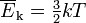﻿ GCI Tech Notes Library

GCI TECH NOTES ©

Volume 15, Number 5
A Gossman Consulting, Inc. Publication
October 2010

## Relative Measurements of Temperature

by

David Gossman

Generally relative measurements of temperatures are expressed on an absolute basis. For example the measured temperature was 200 degrees F +/- 20 degrees F. This is perfectly acceptable. Less often the percentage of a temperature is discussed. In the prior example, at first glance one might express this as 200 degrees F +/- 10%. This would be inaccurate since the Fahrenheit scale is not zeroed but is in fact an offset Rankine scale. If one, for example were to use this concept incorrectly and suggest that an error in measurement was +/- 1% on the Fahrenheit the results would be nonsensical anywhere close to zero, but more importantly they would be nonlinear relative to actual temperature.

At this point it is worth discussing what temperature actually measures. From Wikipedia we have:

“The temperature of a classical ideal gas is related to its average kinetic energy via the equation:where k = R / n (n= Avogadro number, R= ideal gas constant).”

In other words temperature is directly proportional to the average kinetic energy of a system. Of course in this case temperature is on an absolute basis, generally expressed as Kelvin, or in the Fahrenheit system, Rankine.

Given this definition of temperature when we discuss the “percent temperature” or “percentage of a temperature” it is easy to see that we are in fact discussing “percentage of kinetic energy” The only way to accurately determine this is to fist convert any relative temperature scale such as Centigrade or Fahrenheit to their respective absolutes, calculate the percentage on that basis which can then be expressed either using the relative scale or the absolute scale. In other words the “percentage of a temperature should be the same irregardless of whether it is expressed as Fahrenheit or Rankine since both of these scales have the same proportionality to kinetic energy – just a zero offset for Fahrenheit.

Using this information we can now calculate 10% of 400 degrees Fahrenheit. First we convert degrees Fahrenheit to Rankine by adding 459 to obtain 859 degrees Rankine. 10% of  859 is 85.9. Therefore 10% of 400 degrees Fahrenheit is in fact 85.9 degrees Fahrenheit. The alternative erroneous method previously discussed results in 40 degrees Fahrenheit, which while looking right, is nonsensical from both a scientific and mathematical perspective. Looked at in another way this determination would be just as wrong as suggesting that 60 degrees Fahrenheit is twice as warm as 30 degrees Fahrenheit.